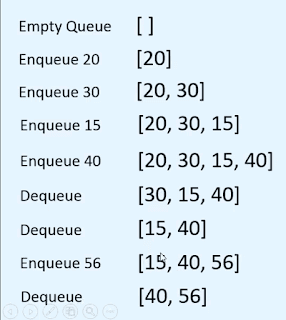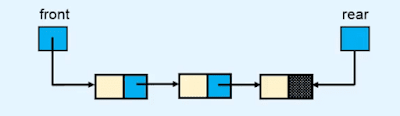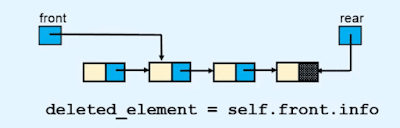A queue is an abstract data structure in which the insertion is performed on one end and deletion is performed on the other end.## Example of Queue in Data structures

In the above-given image, you see that the customers are standing in a line. and the customer who entered the line will go first means First in First out. and we can add the new customers in line at the end of the line and will go out from the beginning of the line.

so the queue follows the FIFO ( First in First out ) process. and we can insert a new value from one end and delete the first inserted value from the other end.

#### Enqueue operation

To insert a new value in the queue is called the Enqueue operation in the queue

#### Dequeue operation

To delete the first entered value in the queue is called the Dequeue operation in the queue.

#### Front end

the end from which we delete the value in the queue is called the Front end of the queue.

#### Rear-end

the end from which we insert a new value in the queue is called the rear end of the queue.if we want to enter a new value then we use the rear end and if we want to delete the value then we use the front end.### Implementation of the queue using the array.to implement a queue using array first we need to choose the position of the front end rear end. we use the first index of array as the front end and last index as rear end.In the above example first, we take an empty array and then we enqueue the values 20, 30, 15 and 40 and after that, we dequeue the value 20, 30 from the front end and then we again enqueue the value 56 and then dequeue the value 15.

#### Program to implement queue using array in a python programming language.

```class EmptyQueueError(Exception):
pass
class Queue:

def __init__(self):
self.items = []

def is_empty(self):
return self.items == []

def size(self):
return len(self.items)

def enqueue(self,item):
self.items.append(item)

def dequeue(self):
if self.is_empty():
raise EmptyQueueError("Queue is Empty")
return self.items.pop(0)

def peek(self):
if self.is_empty():
raise EmptyQueueError("Queue is Empty")
return self.items

def display(self):
print(self.items)

##############

if __name__ == '__main__':
qu = Queue()

while True:
print("1. Enqueue")
print("2. Dequeue")
print("3. Peek")
print("4. Size")
print("5. Display")
print("6. Quit")

choice = int(input("Enter your choice : "))

if choice == 1:
x = int(input("Enter the element : "))
qu.enqueue(x)
elif choice == 2:
x=qu.dequeue()
print("Element deleted fro the queue is : ", x)
elif choice == 3:
print("Element at the front end is ", qu.peek())
elif choice == 4:
print("Size of queue ", qu.size())
elif choice == 5:
qu.display()
elif choice == 6:
break        else:
print("Wrong choice ")
print()```

### Implementation of the queue using the linked listto implement a queue using the linked list we first store the first node and last node's reference into the front end rear variables. because using the front variable we perform deletion in a queue and using the rear variable we perform insertion in a queue.

so the beginning of the list called the front end of the queue and end of the list called the rear end of the queue.

#### Dequeue operation

we already know how to delete the first node of the linked list and delete the node in the only linked list. and if you don't know then please go through this tutorial#### Enqueue operation

we already know how to insert a new node at the end of the linked list. and if you don't know then please go through this tutorial#### Program to implement the queue using the linked list in the python programming language.

```class EmptyQueueError(Exception):
pass

class Node:

def __init__(self, value):
self.info = value

class Queue:

def __init__(self):
self.rear = None
def is_empty(self):
return self.rear is None
def size(self):
if self.is_empty():
return 0        n = 0        p = self.rear.link
while True:
n += 1            p = p.link
break        return n

def enqueue(self, data):
temp = Node(data)

if self.is_empty():
self.rear = temp
else:
self.rear = temp

def dequeue(self):

if self.is_empty():
raise EmptyQueueError("Queue is Empty ")

temp = self.rear
self.rear = None        else:
return temp.info

def peek(self):
if self.is_empty():
raise EmptyQueueError("Queue is Empty")

def display(self):
if self.is_empty():
print("List is empty")
return
while True:
print(p.info, " ", end='')
break        print()

#####################

if __name__ == '__main__':
qu = Queue()

while True:
print("1. Enqueue")
print("2. Dequeue")
print("3. Peek")
print("4. Size")
print("5. Display")
print("6. Quit")

choice = int(input("Enter your choice : "))

if choice == 1:
x = int(input("Enter the element : "))
qu.enqueue(x)
elif choice == 2:
x = qu.dequeue()
print("Element deleted from the queue is : ", x)
elif choice == 3:
print("Element at the front end is ", qu.peek())
elif choice == 4:
print("Size of queue ", qu.size())
elif choice == 5:
qu.display()
elif choice == 6:
break        else:
print("Wrong choice")
print()```